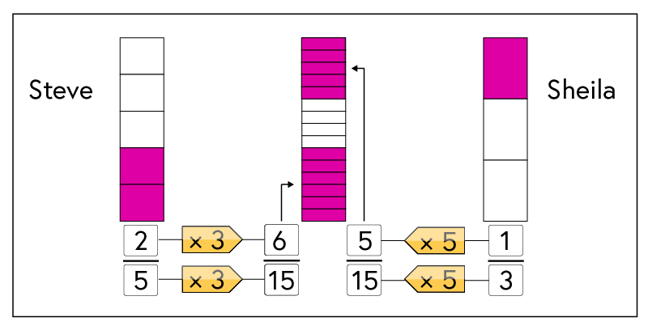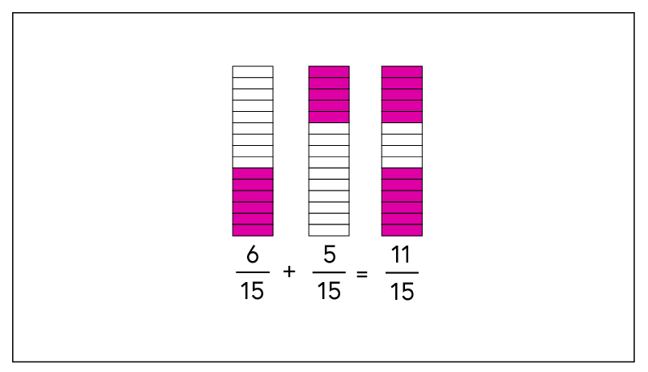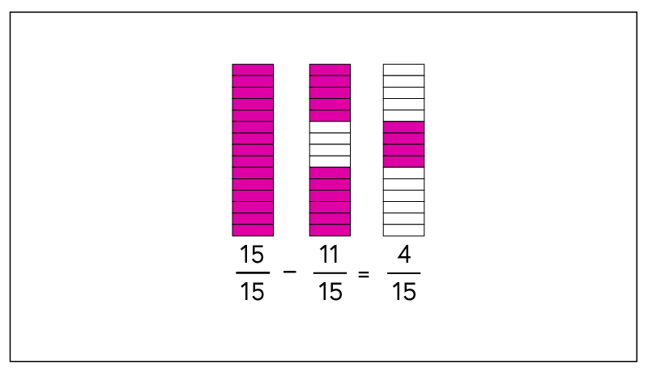## Want to keep learning?

This content is taken from the National STEM Learning Centre's online course, Maths Subject Knowledge: Fractions, Decimals, and Percentages. Join the course to learn more.
4.9

# Worded questions and working with three or more fractions

Sometimes a question written in words can appear more difficult. Written questions require the student to extract the important information, create a mathematical model and identify the type of calculation they are needing to perform.

## Example

Steve and Sheila buy a digital TV recorder. Steve fills $$\frac{2}{5}$$ of the recorder with his programmes and Sheila fills $$\frac{1}{3}$$ of the recorder with her programmes.

a) What fraction of the recorder is full?
b) What fraction of the recorder is empty?

Steve deletes one of his recordings which takes up $$\frac{1}{10}$$ of the recorder.

c) What fraction of the recorder do Steve’s recordings fill now?

From the question we have identified that there are two fractions representing two parts of the same whole, the digital recorder: $$\frac{2}{5}$$ and $$\frac{1}{3}$$.

To answer part a) we need to make the denominators the same:The denominators of our two fractions are 3 and 5, both prime numbers, so we use the lowest common multiple, 15, as our denominator.

To get our equivalent fractions of fifteenths, we multiply the numerator and denominator of each fraction as shown above. $$\frac{2}{5}$$, multiplying top and bottom by 3, becomes $$\frac{6}{15}$$ and $$\frac{1}{3}$$, multiplying top and bottom by 5, becomes $$\frac{5}{15}$$.

We can now add the two fractions together as they have the same denominator:$\frac{6}{15} + \frac{5}{15} = \frac{11}{15}$

This means, $$\frac{11}{15}$$of the recorder is full.

b) To find how much of the recorder is empty, we know that the ‘whole’ recorder can be represented as ‘1’, and this can be represented as $$\frac{15}{15}$$ (using the denominator of our previous answer). It’s then straightforward to take away $$\frac{11}{15}$$ from $$\frac{15}{15}$$, to get $$\frac{4}{15}$$. Alternatively we could say that the difference between $$\frac{15}{15}$$ and $$\frac{11}{15}$$ is $$\frac{4}{15}$$, so $$\frac{4}{15}$$ of the disc is empty.c) The final part of the question asks us to take $$\frac{1}{10}$$ of $$\frac{2}{5}$$ away from $$\frac{2}{5}$$.

First we find the equivalent fraction of $$\frac{2}{5}$$ with 10 as the denominator. This gives $$\frac{4}{10}$$.

Now we take $$\frac{1}{10}$$ away from $$\frac{4}{10}$$ to get $$\frac{3}{10}$$.

Hence, Steve’s recordings now take up $$\frac{3}{10}$$ of the recorder.

## Discuss

Work through this question again, where are the points in this question where students might make a mistake? Can you come up with any general advice for tackling worded questions? Share in the comments below.

## Problem worksheet

Now complete questions 9, 10 and 11 from this week’s worksheet.
As a reminder, the worksheet can be found in the first step of this week.